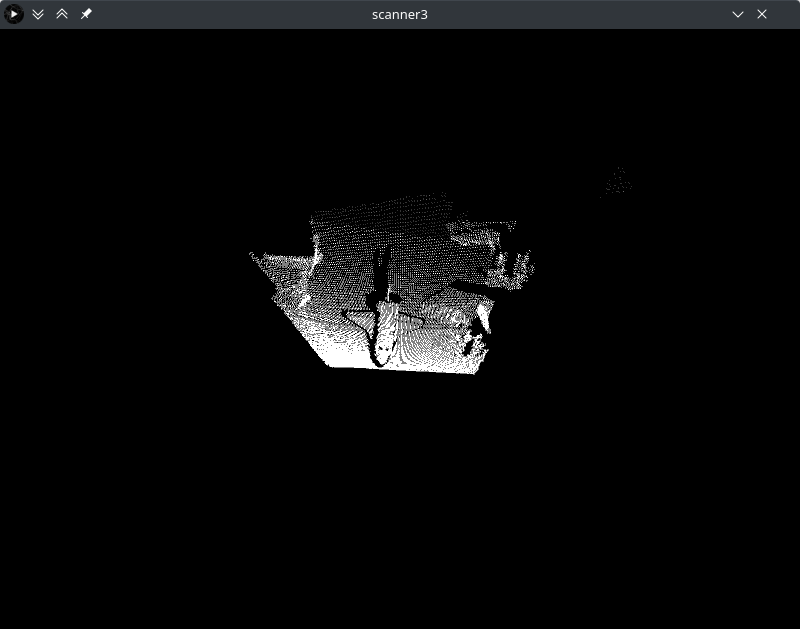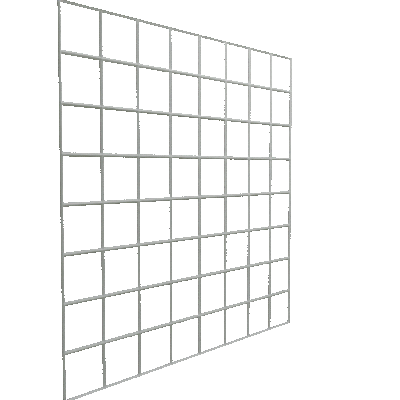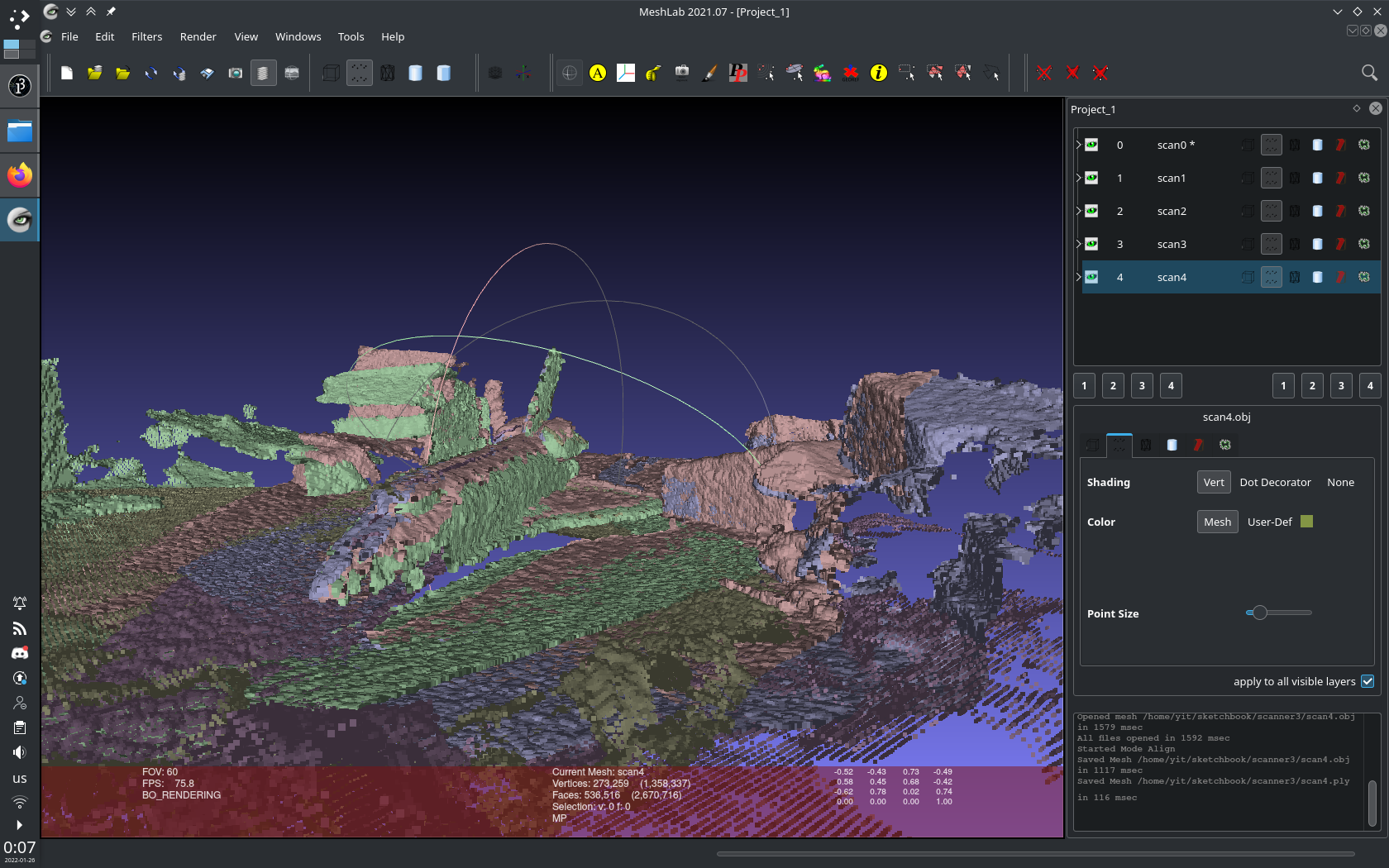# 3d scanning with an xbox kinect

I have always wanted to do some 3d scanning, but I couldn't get photogrammetry set up on my computer. Recently I got a kinect, a camera that also does depth so it should be able to do 3d scanning. Processing seemed like a good choice for a language to use, since I am familiar with it and it has a kinect library. My code is directly descended from the point cloud example for openkinect-for-processing.

The first step after getting the depth values from the kinect is to undo the perspective distortion. A small change in position in points that are close to the kinect have a much larger change in apparent position than points that are far from the kinect. The basic solution to this is to multiply the `x` and the `y` by the `z`.Now that there is a point cloud of world coordinates, it just has to be saved to a file. The format I chose is obj, since it is so easy to output from a program. To put a vertex in an obj file, just put a line in the file with `v x y z` where `v` is the letter v, and `x y z` are the x, y, and z coordinates of the vertex. After all this is implemented, the program is capable of 3d scanning.All you have to do is take multiple scans from different angles, then remove the unwanted points, then match them up (I used blender to do this), and the turn the points into a mesh (I used meshlab to do this). The problem with this approach is that it is a lot of effort, particularly the lining up the point clouds. I found out that meshlab is able to line up multiple partial scans as long as they are a mesh. I had two ideas. I could either use meshlab to mesh all the point clouds, and then match them up, or I could mesh the scans in the program I wrote. All the points in the point cloud come from the kinect, which captures them as a grid. This allows us to take the grid, and keep the faces how they were, even after transforming them with the depth.The next thing to automate is remove the unwanted points. I chose to do this by filtering the depth. Anything more than 1.5 meters I filtered out, since it is probably the background. Additionally I filtered out the points with a depth of zero, since that means that their depth did not get recorded correctly. This creates two problems. The easy one is that some faces will no longer have all the vertices, so I just got rid of those faces. The hard one is that when the vertices get deleted, the indices of all the following ones change. What I originally had in the code was to add all the vertices, and then the indices for the faces would follow a simple `x + y*width` formula. Alternatively, in pseudocode:

```for x from 0 to width:
for y from 0 to height:
add vertex x, y, z to obj file
for x from 0 to width-1:
for y from 0 to height-1:
add face x+y*width (x+1)+y*width (x+1)+(y+1)*width x+(y+1)*width obj file```

My solution to recalculating the indices is for the face to store a reference to the vertices, and turn it to a number after the unnecessary vertices get deleted. pseudocode:

```for x from 0 to width:
for y from 0 to height:
append to vertices vertex x, y, z

for x from 0 to width-1:
for y from 0 to height-1:
append to faces face(
vertex a = get vertex x+y*width
vertex b = get vertex (x+1)+y*width
vertex c = get vertex (x+1)+(y+1)*width
vertex d = get vertex x+(y+1)*width
)

for every vertex
if the depth is not in range, get rid of it

index = 0

for every remaining vertex